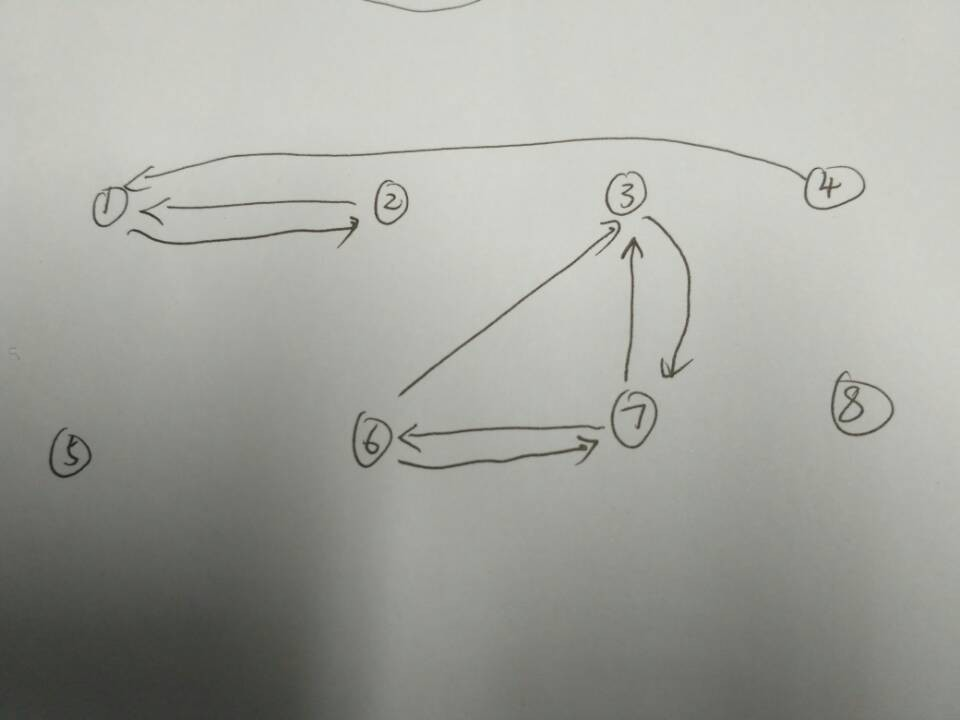# Spark组件之GraphX学习14--TriangleCount实例和分析

1解释

/*
* Licensed to the Apache Software Foundation (ASF) under one or more
* contributor license agreements.  See the NOTICE file distributed with
* this work for additional information regarding copyright ownership.
* The ASF licenses this file to You under the Apache License, Version 2.0
* (the "License"); you may not use this file except in compliance with
* the License.  You may obtain a copy of the License at
*
*    http://www.apache.org/licenses/LICENSE-2.0
*
* Unless required by applicable law or agreed to in writing, software
* distributed under the License is distributed on an "AS IS" BASIS,
* WITHOUT WARRANTIES OR CONDITIONS OF ANY KIND, either express or implied.
* See the License for the specific language governing permissions and
* limitations under the License.
*/

package org.apache.spark.graphx.lib

import scala.reflect.ClassTag

import org.apache.spark.graphx._

/**
* Compute the number of triangles passing through each vertex.
*
* The algorithm is relatively straightforward and can be computed in three steps:
*
* <ul>
* <li>Compute the set of neighbors for each vertex
* <li>For each edge compute the intersection of the sets and send the count to both vertices.
* <li> Compute the sum at each vertex and divide by two since each triangle is counted twice.
* </ul>
*
* Note that the input graph should have its edges in canonical direction
* (i.e. the sourceId less than destId). Also the graph must have been partitioned
* using [[org.apache.spark.graphx.Graph#partitionBy]].
*/
object TriangleCount {

def run[VD: ClassTag, ED: ClassTag](graph: Graph[VD, ED]): Graph[Int, ED] = {
// Remove redundant edges
val g = graph.groupEdges((a, b) => a).cache()

// Construct set representations of the neighborhoods
val nbrSets: VertexRDD[VertexSet] =
g.collectNeighborIds(EdgeDirection.Either).mapValues { (vid, nbrs) =>
val set = new VertexSet(4)
var i = 0
while (i < nbrs.size) {
// prevent self cycle
if (nbrs(i) != vid) {
set.add(nbrs(i))
}
i += 1
}
set
}
// join the sets with the graph
val setGraph: Graph[VertexSet, ED] = g.outerJoinVertices(nbrSets) {
(vid, _, optSet) => optSet.getOrElse(null)
}
// Edge function computes intersection of smaller vertex with larger vertex
def edgeFunc(ctx: EdgeContext[VertexSet, ED, Int]) {
assert(ctx.srcAttr != null)
assert(ctx.dstAttr != null)
val (smallSet, largeSet) = if (ctx.srcAttr.size < ctx.dstAttr.size) {
(ctx.srcAttr, ctx.dstAttr)
} else {
(ctx.dstAttr, ctx.srcAttr)
}
val iter = smallSet.iterator
var counter: Int = 0
while (iter.hasNext) {
val vid = iter.next()
if (vid != ctx.srcId && vid != ctx.dstId && largeSet.contains(vid)) {
counter += 1
}
}
ctx.sendToSrc(counter)
ctx.sendToDst(counter)
}
// compute the intersection along edges
val counters: VertexRDD[Int] = setGraph.aggregateMessages(edgeFunc, _ + _)
// Merge counters with the graph and divide by two since each triangle is counted twice
g.outerJoinVertices(counters) {
(vid, _, optCounter: Option[Int]) =>
val dblCount = optCounter.getOrElse(0)
// double count should be even (divisible by two)
assert((dblCount & 1) == 0)
dblCount / 2
}
} // end of TriangleCount
}


outerJoinVertices

2.代码：

/**
* @author xubo
* ref http://spark.apache.org/docs/1.5.2/graphx-programming-guide.html
* time 20160503
*/

package org.apache.spark.graphx.learning

import org.apache.spark.SparkConf
import org.apache.spark.SparkContext
import org.apache.spark.graphx.Graph
import org.apache.spark.graphx.Graph.graphToGraphOps
import org.apache.spark.graphx.VertexId
import org.apache.spark.graphx.util.GraphGenerators
import org.apache.spark.graphx.GraphLoader
import org.apache.spark.graphx.PartitionStrategy

object TriangleCounting {

def main(args: Array[String]): Unit = {
val conf = new SparkConf().setAppName("ConnectedComponents").setMaster("local")
val sc = new SparkContext(conf)

// Load the edges in canonical order and partition the graph for triangle count
val graph = GraphLoader.edgeListFile(sc, "file/data/graphx/input/followers.txt", true).partitionBy(PartitionStrategy.RandomVertexCut)
// Find the triangle count for each vertex
val triCounts = graph.triangleCount().vertices
// Join the triangle counts with the usernames
val users = sc.textFile("file/data/graphx/input/users.txt").map { line =>
val fields = line.split(",")
(fields(0).toLong, fields(1))
}
val triCountByUsername = users.join(triCounts).map {
case (id, (username, tc)) =>
(username, tc)
}
// Print the result
println("\ngraph edges");
println("edges:");
graph.edges.collect.foreach(println)
graph.edges.collect.foreach(println)
println("vertices:");
graph.vertices.collect.foreach(println)
println("triplets:");
graph.triplets.collect.foreach(println)
println("\nusers");
users.collect.foreach(println)

println("\n triCounts:");
triCounts.collect.foreach(println)
println("\n triCountByUsername:");
println(triCountByUsername.collect().mkString("\n"))

}
}3.结果：

graph edges
edges:
Edge(1,2,1)
Edge(1,2,1)
Edge(1,4,1)
Edge(3,6,1)
Edge(3,7,1)
Edge(3,7,1)
Edge(6,7,1)
Edge(6,7,1)
Edge(1,2,1)
Edge(1,2,1)
Edge(1,4,1)
Edge(3,6,1)
Edge(3,7,1)
Edge(3,7,1)
Edge(6,7,1)
Edge(6,7,1)
vertices:
(4,1)
(6,1)
(2,1)
(1,1)
(3,1)
(7,1)
triplets:
((1,1),(2,1),1)
((1,1),(2,1),1)
((1,1),(4,1),1)
((3,1),(6,1),1)
((3,1),(7,1),1)
((3,1),(7,1),1)
((6,1),(7,1),1)
((6,1),(7,1),1)

users
(1,BarackObama)
(2,ladygaga)
(3,jeresig)
(4,justinbieber)
(6,matei_zaharia)
(7,odersky)
(8,anonsys)

triCounts:
(4,0)
(6,1)
(2,0)
(1,0)
(3,1)
(7,1)

triCountByUsername:
(justinbieber,0)
(matei_zaharia,1)
(ladygaga,0)
(BarackObama,0)
(jeresig,1)
(odersky,1)

 triCounts:
(4,1)
(6,1)
(2,1)
(1,1)
(3,1)
(7,1)

triCountByUsername:
(justinbieber,1)
(matei_zaharia,1)
(ladygaga,1)
(BarackObama,1)
(jeresig,1)
(odersky,1)

【1】 http://spark.apache.org/docs/1.5.2/graphx-programming-guide.html